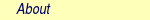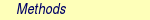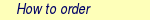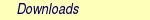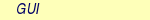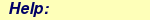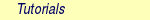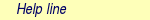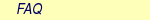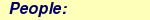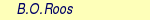MOLCAS manual:Next: 8.39 quater Up: 8. Programs Previous: 8.37 poly_aniso

Subsections

# 8.38 qmstat

## 8.38.1 Description

QmStat couples a quantum chemical region to a statistically mechanically described surrounding thus creating an effective Hamiltonian for the quantum chemical region Heff.. This way solvent effects can be accounted for.

The surrounding is discrete in contrast to the continuum models, such as PCM (also available in MOLCAS see Seward). The explicit representation of the solvent enables a more accurate description of the solvation, but also makes the model more complex and significantly less black-box''. For example, the interaction within the statistical mechanical surrounding has to be accounted for with an accurate enough force-field. In its present implementation QmStat only treats water as described by an early version of NEMO, which includes polarization of the molecules . Also, the interaction between the quantum chemical region (typically the solute) and the surrounding (typically the solvent) has to be considered in more detail than in a continuum model. Several approaches to discrete (or explicit) solvation are thus possible. The approach in QmStat is summarized below, see also [73,74,75,76].

To include entropic effects to the solvation phenomena, QmStat uses the Metropolis-Monte Carlo simulation technique. This means that random steps are taken in the space of solute-solvent configurations, some of which are accepted, others rejected, on account of the usual energy difference criteria. This implies that at each step, an energy has to be evaluated. Using normal quantum chemical methods, this is usually too restrictive, since roughly one million Monte Carlo steps are required to converge the statistical mechanical treatment. QmStat proceeds by doing simplifications to the quantum chemistry, not the statistical mechanics, as is the more common way forward. QmStat is therefore a hybrid QM/MM methods (according to one existing terminology).

Two simplified quantum chemical methods are presently available: orbital basis Hartree-Fock and a state basis formulation, which is approximate to the CASSCF method. Both formulations uses the fact that there is only minor differences in the electronic structure for the different configurations in the Monte Carlo simulation. Therefore, a basis as general as the standard atomic orbital basis sets is redundant. QmStat constructs either a more compact orbital basis or a more compact basis in terms of states to expand the solvated wave function. This requires some work before the simulation, but has the advantage that during the simulation, less computational work is needed.

Finally, a comment on the way the energy is computed for a given configuration is needed. The evaluation of the interactions between the solvent molecules is prescribed by the construction of the force-field and are relatively simple. The interaction between the quantum chemical region and the solvent is formulated to include electrostatic and non-electrostatic interactions. The former is described in a multi-center multipole expanded way, while the latter models the effect the antisymmetry principle between solute and solvent electrons has on the solute electronic structure. Its formulation is similar to pseudo-potentials. Also a phenomenological term for the dispersion is added. Long range electrostatics, finally, is described with a dielectric cavity model.

## 8.38.2 Dependencies

The dependencies of QmStat differ for the two quantum chemical methods. In the Hartree-Fock description, Seward, FfPt, Scf, Averd, MpProp and Motra typically have to precede. If an orbital basis is taken from somewhere else FfPt, Scf and Averd are not mandatory. For the RASSI alternative, typically Seward, Scf, RasScf, MpProp and Rassi precede QmStat.

## 8.38.3 Files

Below is a list of the files that are used/created by the program QmStat.

### 8.38.3.1 Input files

 File Contents ONEINT One-electron integral file generated by the program SEWARD. RUNFILE File for communication of auxiliary information generated by the program SEWARD. RUNFILEW File for communication of auxiliary information generated by the program SEWARD for the solvent molecule. AVEORB (Only for Hartree-Fock alternative). Average orbitals generated by AVERD. If other orbitals are to be used, they should be given the above name; in other words, the orbitals must not be created by AVERD, it is only customary. SOLORB Solvent orbitals generated by SCF. TRAONE (Only for Hartree-Fock alternative). Molecular orbital transformed one-electron integrals generated by MOTRA. TRAINT (Only for Hartree-Fock alternative). Molecular orbital transformed two-electron integral generated by MOTRA. MPPROP File generated by MPPROP. DIFFPR Exponents and Prefactors for a Slater desciption of the Electrostatics to take into account the penetration effects due to the overlap.File generated by MPPROP. RASSIM (Only for the RASSI alternative). The transition density matrix generated by RASSI. The keyword TOFILE has to be given in the input to RASSI. EIGV (Only for the RASSI alternative). Information about the eigenvectors and their energy generated by RASSI (TOFILE needed). ADDON* File with additional one-electron perturbation to be added to the Hamiltonian matrix. This file is only required if EXTERNAL is used.

### 8.38.3.2 Output files

 File Contents STFIL* Start files in which solvent configurations are stored at intervals during the simulation. They enable the simulation to restart, hence they can also be as input to Qmstat. SAFIL* Sampling files in which a selection of configurations are stored for analysis. They can in some applications also act as input to Qmstat, usually in free-energy perturbation calculations. EXTRA* Extract files which are formatted files in which data from the analysis of the sampling files are stored.

## 8.38.4 Input

The complexity inherit in a discrete solvent model engenders a, potentially, complex input. To (hopefully) make the input transparent the set of keywords are ordered in several tiers. Below all keywords and their sub- and subsubkeywords are presented. A keyword with several tiers should typically be of the following form

SIMUlation
...(keywords  on  higher  tier)
END  simulation
Also consult the input example below and the examples in section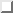for guidance. Mandatory keywords are highlighted.

 Keyword Meaning TITLe Title to the calculation. SIMUlation Keywords relating to the how the simulation is to be performed and under which conditions. RADIus Initial radius of the dielectric cavity. The radius is also specified on the startfile and has higher priority than the radius given with the present keyword. PERMittivity Permittivity of the dielectric continuum. 80 on default. TEMPerature Temperature in Kelvin. Default is 300. PRESsure Macroscopic pressure in atmosphere. Default is 1 atm. SURFace Surface tension parameter for the cavity. Default is for air-water interface. TRANslation Maximal translation in the simulation (in a.u. )Default is 0.0 a.u. ROTAtion Maximal angle for rotation of solvent around molecular axes. Default is 0o. CAVIty Maximal modification of radius of dielectric cavity. Default is 0.0 a.u. FORCe Force constant for the harmonic potential that presents a bias in the simulation for configurations with the QM-region close to the center of the cavity. Default is 0.001. BREPulsion Parameter for the Repulsion energy that keeps the QM-region away from the boundary. Default is 0.0 a.u. SEED Seed to the pseudo-random number generator. PARAlleltemp A parallel tempering procedure is performed to boost sampling. It is mainly used in systems with small transition elements in the Markov chain, which will give difficult samplings. Three lines follow: First line gives the number of different temperatures to perform the simulation, NTemp. In the second line Ntemp integers are given, each of these specify a file to store the configuration for each temperature. Third line gives the NTemp temperatures used for the tempering procedure. END_Simulation Parameters Marks the end of the input to the simulation parameters. THREshold Followed by three numbers. First the threshold for the induced dipoles in the generalized self-consistent field method for the solution of the mutual polarization problem is specified. Second the the threshold for the energy in the same method is given. Finally the maximum number of iterations in the method is specified. Defaults are 0.0001 0.0000001 and 30. STEPs Followed by two entries. Number of macrosteps and number of microsteps. The total number of steps is the product of the two numbers above. At the end of each macrostep the relevant STFIL is up-dated. Default is 1 and 1. RUN Specify type of simulation. 'QMEQ' means quantum chemical equilibration; only the startfile is up-dated. 'QMPR' means quantum chemical production; startfile is up-dated and sampfile constructed. Observe that if 'QMPR' is specified a line with two entries follows in which the interval of sampling is specified and on which sampfile (1-7) the data is to be stored. 'ANAL' means an analysis of the stored results is to be performed. PRINt Print level. 1 is default and anything above this number can generate large outputs. No higher than 10 is recommended for non-developers. EXTErnal An external perturbation is to be added to the Hamiltonian in the Rassi alternative. The arguments are number of perturbation matrices, N, followed by N lines. Each line has the form: ci a scalar with which the perturbation can be scaled, Vi is a character string with the label of the perturbation as given on SEWARD's one-electron integral file, nci is the component number of the perturbation. A final expression for the perturbation would be: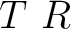. CONFiguration Keywords relating to from which source the initial solvent configuration is to be obtained. It is mandatory to specify a source. ADD Followed by one number specifying how many solvent molecules that are to be added at random to the cavity. This is the worst way to start a simulation since it will take a lot of time to equilibrate the system. FILE Signify that start configuration is to be read from some file. STARtfile Read solvent configuration from startfile. SCRAtch Read solvent configuration from startfile and place the QM-region as given on RUNFILE. COPY Read solvent and QM configuration from startfile. This is he keyword to use if a simulation is to be restarted. Observe that consistent startfile and RUNFILE must be used. CM__ Read solvent configuration from startfile and place the QM in the center of mass of the QM placed on startfile. For any of the previous keywords two numbers are given, Nin and Nout which specify from which startfile QmStat is supposed to read and write, respectively SAMPfile Read solvent configurations put on a sampfile and analyze them. Two numbers are given, Nin and Nextr which specify from which sampfile QmStat is supposed to read and on which extract file the results are to be put. INPUt The starting configuration is to be read from the input. The coordinates are given after the keyword COORdinates in the second tier to the SOLVent keyword. One number as argument: the startfile to which configurations are written. END_Configuration Marks the end of the input to the initial configuration. EDIT Signify that a startfile is to be edited. If this keyword is given, then no simulation will be performed. DELEte Two rows follow; on the first Nin and Nout are given which specify the startfile to read from and write to, respectively; on the second the number of solvent molecules to delete. The solvent molecules farthest away from origin are deleted. ADD The form of the arguments as DELEte above, only the second row give number of molecules to add. Observe that the keyword RADIus will with the present keyword specified give the radius of the cavity of the edited startfile. QMDElete Delete the QM-region and substitute it by water molecules. One row follows with two numbers, which specify the startfile to read from and write to, respectively. DUMP Dump startfile coordinates in a way suitable for graphical display. Two rows follow; on the first a character string with the format the coordinated will be dumped; on the second Nin specifies the startfile to read. Currently there is only one format for this keyword: MOLDen. END_EditStartFile Marks the end of the input to edit the startfile. QMSUrrounding Keywords that are related to the interaction between surrounding and the quantum chemical region. DPARameters Parameters for the dispersion interaction. Follow N lines, which N the number of atoms in the QM-region. The general form for each line is: d1 and d2 where d1 is the dispersion parameter between one atom of the QM-region and the water oxygen, and d2 is the same but regarding to the hydrogen of the water.The order of the QM atoms is given by RUNFILE. ELECtrostatic Parameters to describe the electrostatic penetration using Slater integrals. THREsholds Two number follow. First, the cutoff (distance Quantum Site-Classical molecule) to evaluate penetration effects. Default is 6 a.u. Second, difference between two Slater exponents to not be consider the same value. Default is 0.001. NOPEnetration No electric penetration is considered in the calculations. Penetration is considered by default. QUADrupoles Electrostatic Penetration computed in quadrupoles. Default is that penetration is computed up to dipoles. END Electrostatic Marks the end of the input to the electrostatic penetration computed by Slater. XPARameters Parameters to describe the repulsion energy. S2 The parameter for theterm. Default zero. S4 The parameter for the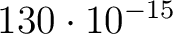term. Default zero. S6 The parameter for the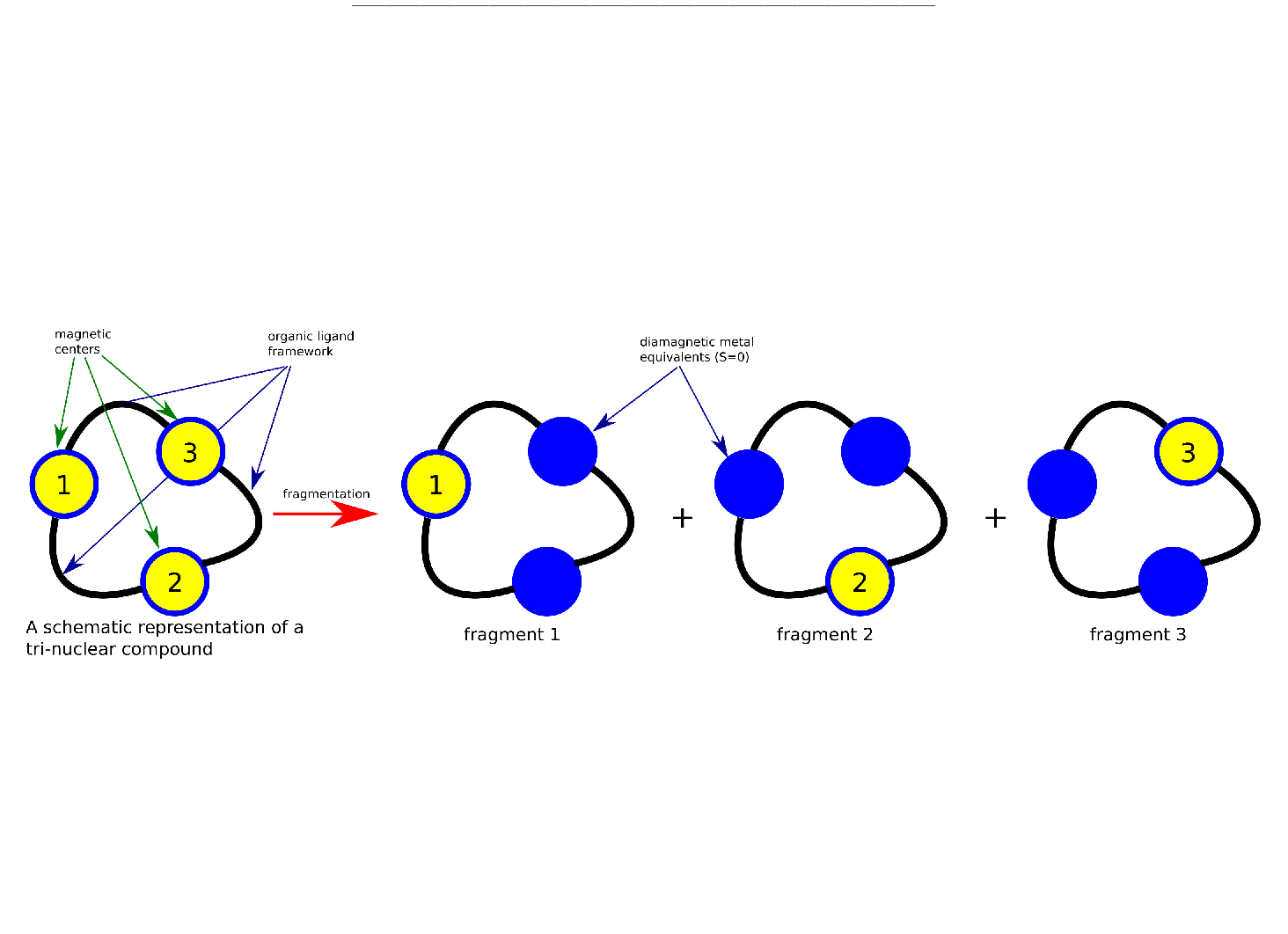term. Default zero. S10 The parameter for the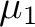term. Default zero. CUTOff Two numbers follow. The first is the cut-off radius such as if any distance from the given solvent molecule is longer than this number, the overlap term is set to zero. The second is a cut-off radius such as if any distance from the given solvent molecule is shorter than this number the energy is set to infinity, or practically speaking, this configuration is rejected with certainty. Defaults are 10.0 a.u. and 0.0 a.u. END XParameters Marks the end of the input to the repulsive parameters. DAMPing DISPersion Input parameters to a dispersion damping expression. The parameters are number obtain from a quantum chemical calculation. All lines have the form: Cval, Qxx, Qyy, Qzz where Cval is the valence charge and Q** are diagonal terms in the quadrupole tensor. First two lines are for the hydrogen atom then the oxygen atom in a water molecule. Next follows as many lines as atoms in the QM region. All these quantities can be obtained from a calculation with MpProp. The numbers are given as input so that the user can if it is found to be needed, modify the damping. Default is no damping. The order of the atoms in the QM region is given by RUNFILE. FIELd The electric field between QM region and surrounding is damped. Three numbers are arguments:CO, CH, N where they are parameters to a field damping expression (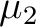) where x is O if the point in the surrounding is on a oxygen atom, H if on a hydrogen atom; R is the distance between the point in the QM region and the points in the surrounding. END Damping Marks the end of the input to the Damping parameters. END QmSurrounding Marks the end of the input related to the interaction between surrounding and the quantum chemical region. SOLVent Keywords that govern the solvent-solvent interaction and some other initial data. Most of these numbers are presently fixed and should not be altered. COORdinates If solvent coordinates are to be given explicitly in input. First line gives number of particles to add. Then follows three times that number lines with coordinates for the oxygen atom and the hydrogen atoms. If the keyword SINGle-point has been given the present keyword assumes a different meaning (see description of SINGle-point). CAVRepulsion Two parameters that regulate the repulsion with the boundary of the cavity. Defaults are 30.0 and 0.06. ATCEchpol Five numbers follow: number of atoms, centers, charges, polarizabilities and slater sites. Defaults are 3, 5, 4, 3 and 5, respectively. CHARge Four numbers follow: the partial charge on the hydrogen atoms and the partial charge on the pseudo-centers. POLArizability Three numbers follow: the polarizability on the oxygen atom and on the two hydrogen atoms. SLATer Magnitude of Slater Prefactors and exponents. One mumber follow: 0 is slater description of electrostatics up to charges, 1 up to dipoles. Then it follows N times (where N is the number of Slater centers) three lines if description up to charge. First line Slater exponent for charges, second line Slater Prefactor and third line nuclear charge of the center. If the description goes up to dipole, N times five lines follows. First two lines are the same as charge description, third line is Slater exponent for dipole, fourth line is the three Slater Prefactors for the dipole (one for each cartesian coordinate) and fith line is the nuclear charge of the center. Defaults: See papers of Karlstrom. If the number of Slater sites is modified this keyword should be after ATCEchpol END Solvent Marks the end of the input that govern the solvent-solvent interaction. RASSisection This section provides the information needed to perform QMSTAT calculations using the RASSI-construction of the wave function. JOBFiles First number give the number of JOB-files that was generated by RasScf (i. e. how many RASSCF calculations that preceded QmStat). The following numbers (as many as the number of JOB-files) specify how many states each calculation rendered. So for example if a State-Average (SA) RASSCF calculation is performed with two states, the number should be 2. EQSTate Which state interacts with the surrounding. Should be 1 if it is the ground state, which also is the default. MOREduce A Reduction of the Molecular Orbitals is performed. One number as argument: the threshold giving the value of the lowest occupation number of the selected natural orbitals . CONTract The RASSI state basis are contracted. One number as argument: the threshold giving the value of the lowest RASSCF overlap for the RASSI state basis . LEVElshift Introduce levelshift of RASSI states. Three lines must be written. First line gives the number of levelshifts to perform. Then follows the states to levelshift (as many as the number of levelshifts). Finally, the value of the levelshift for each state is given. CISElect The QM solvent overlap is used as the criterion to choose the state that interacts with the surrounding. Three lines follow. One entire: among how many states can be chosen the interacting state, N. The second line, N entries giving the number of each state. Finally, N scaling factors, one for each state, of the overlap. END RassiSection Marks the end of the input that govern the Rassi calculations. SCFSection This section provides additional information to perform QMSTAT calculations using the SCF-construction of the wave function. ORBItals Two numbers are required: how many orbitals that are to be used how many occupied orbitals there are in the QM region. as a basis in which to solve the Hartree-Fock equation, and END ScfSection Marks the end of the input that govern the Scf calculations. SINGle-point This keywords signals that a set of single point calculations should be performed; this is typically what one needs when fitting parameters. The keyword gives the COORdinates keyword in the SOLVent section a new meaning. The first row then gives the number of points in which a single-point calculation should be performed and the coordinates that follow give the coordinates for the water monomer. QmStat then run each solute-monomer solvent configuration specified and the energy (among other things) is computed. The keyword thus overrides the usual meaning of the input. Observe that the permittivity has to be set to 1 if one attempts to reproduce a quantum chemical supermolecular potential. EXTRact Section Give details about the analysis performed to the results stored in the sampfile. TOTAl energy The total energy of the whole system is extracted. DIPOle The three components and the total dipole of the QM-region are extracted. QUADrupole The six components and the quadrupole of the QM-region are extracted. EIGEn The Eigenvalues of the RASSI matrix and the eigenvectors are extracted. Follow by a number and a "YES" or "NON" statement. The number gives the highest state where the eigenvalue is extracted. YES means that also the corresponding eigenvectors are extracted. EXPEctation values The expectation values of H0 and main perturbations: Vel, Vpol and Vn-el are extracted. If keyword EIGEn is specified it is done for the same states as this keyword, otherwise the extraction is performed for the equilibrated state. Observe that the expectation values are for the final wave function of the QM-region in solution, so H0 is not the same as for the isolated QM-region. ELOCal The local expectation values of Vel and Vpol for the multipole expansion sites are extracted. Two lines follow. First, gives for how many sites these values will be extracted, N. Second line, N entries giving the number of each site. If keyword EIGEn is specified the extraction is done for the same states as this keyword, otherwise it is performed for the equilibrated state. MESP The Main Electrostatic potential, field and field gradients will be obtained in order to produce perturbation integrals that will be used to optimize the intramolecular geometry of the QM system. Observe that this keyword will change the one electron integrals file, so it is advised to make a copy of the original file. After running this option ALASKA and SLAPAF must be running with the new one electron integrals file in order to produce the gradients and a new geometry in the geometry optimization procedure. END ExtractSection Marks the end of the input that give details about the analysis performed.

### 8.38.4.1 Input example

The following input uses the Rassi alternative and restarts from startfile.0 and write to startfile.1 every 1000th step, where the total number of steps is 200*1000. A set of parameters are given which are for an organic molecule with one carbon, one oxygen and two hydrogen atoms. The order in the previous SEWARD and RASSCF calculations for the atoms is carbon, oxygen, hydrogen 1 and hydrogen 2. The dispersion is damped. Finally, there are sixteen RASSCF calculations preceeding and the last two are state-average since two states are collected from these files; the ground state interacts with the surrounding.

&QmStat  &End

Simulation  *  Simulation  parameters.
Translation
0.03  *  Maximun  translation  step  of  water.
Rotation
1.0  *  Maximun  rotation  step  of  water.
Cavity
0.05  *  Maximun  variation  of  the  cavity  radius  for  step.
End  Simulation

Steps  *  Number  of  macro  and  microsteps.
200  1000

Configuration  *  How  the  start  configuration  is  readed.
Start  *  The  cordinates  are  taken  form  a  startfile.
Copy  *  The  coordinates  of  the  QM  region  are  the  same  as  in  the  startfile.
0  1
End  Configuration

QmSurrounding
DParameters  *  Dispersion  parameters.
35.356  4.556  *  Carbon_QM-Oxygen_wat  Carbon_QM-Hydrogen_wat.
16.517  2.129  *  Oxygen_QM-Oxygen_wat  Oxygen_QM-Hydrogen_wat.
10.904  1.405  *  Hydrogen1_QM-Oxygen_wat  Hydrogen1_QM-Hydrogen_wat.
10.904  1.405  *  Hydrogen2_QM-Oxygen_wat  Hydrogen2_QM-Hydrogen_wat.

XParameters  *  QM-Solvent  Repulsion  Parameters.
S2
-0.375
S6
1.7
End  XParameters
Damping  *  Dispersion  Damping.
Dispersion
-6.64838476  -5.22591434  -4.32517889  -4.58504467  *  Water  Hydrogen.
-.34146881  -0.21833165  -0.22092206  -0.21923063  *  Water  Oxygen.
-4.23157193  -1.91850438  -2.28125523  -1.91682521  *  Quamtum  Carbon.
-6.19610865  -3.90535461  -4.73256142  -3.77737447  *  Quantum  Oxygen.
-.57795931  -0.42899268  -0.43228880  -0.43771290  *  Quantum  Hydrogen  1.
-.57795931  -0.42899268  -0.43228880  -0.43771290  *  Quantum  Hydrogen  2.
End  Damping
End  QmSurrounding

RassiSection
JobFiles  *  Number  of  JobFiles.
16
1  1  1  1  1  1  1  1  1  1  1  1  1  1  2  2  *  One  state  is  collected  form  all  JobFiles
*  except  from  the  two  last  ones,  which  two
*  are  collected.
EqState  *  The  state  interacting  with  the  surrounding.
1
End  RassiSection

End  of  Input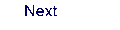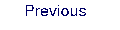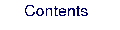Next: 8.39 quater Up: 8. Programs Previous: 8.37 poly_aniso